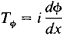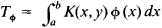(redirected from Hermitian operators)

[¦self ə¦jȯint ′äp·ə‚rād·ər]
(mathematics)
A linear operator which is identical with its adjoint operator.
McGraw-Hill Dictionary of Scientific & Technical Terms, 6E, Copyright © 2003 by The McGraw-Hill Companies, Inc.
The following article is from The Great Soviet Encyclopedia (1979). It might be outdated or ideologically biased.

(or Hermitian operator), an operator coincident with its adjoint. The theory of self-adjoint operators arose as a generalization of the theory of, for example, integral equations with a symmetric kernel, self-adjoint differential equations, and symmetric matrices. Examples of self-adjoint operators are (1) the operator of multiplication by the independent variable in the space of functions that are defined on the entire number axis and are square integrable and (2) the differentiation operatorin the same space.

If the function K(x,y) is continuous in the square a ≤ x ≤ b,a ≤ y ≤ b and if K(x,y) = K(y, x), then the integral operatoris self-adjoint. The spectrum of a self-adjoint operator lies on the real axis. In quantum mechanics physical quantities have corresponding self-adjoint operators whose spectra give the possible values of these quantities. A self-adjoint operator can be represented as an integral that is the limit of linear combinations of pairwise orthogonal projection operators with real coefficients.

References in periodicals archive ?
Hermitian Operators on a Banach Algebras of Continuous Maps Whose Values Are in a Uniform Algebras
The [d.sup.2] phase point operators [A.sub.[alpha]] are linearly independent and form a basis for the space of Hermitian operators acting on a d-dimensional Hilbert space.
Lee, Maps preserving zero Jordan products on hermitian operators, Illinois J.
I came across the book on several occasions, e.g., when reading about the directed order structure for families of hermitian operators, e.g., for those whose limit is the sup [6, p.
He explains vector spaces and bases, linear transformations and operators, eigenvalues, circles and ellipses, inner products, adjoints, Hermitian operators, unitary operators, the wave equation, continuous spectre and the Dirac delta function, Fourier transforms, Green's and functions, and includes an appendix on matrix operations (new to this edition) and a full chapter on crucial applications.
KREIN, Fundamental aspects of the representation theory of Hermitian operators with deficiency index (mrz, rn), Ukrain.
The way in which physical concepts are tied to the mathematical formalism is particularly helpful, for example in the treatment of Hermitian operators where orthogonality of non-degenerate eigenstates is tied to repeatability of measurements.
Properties of such systems are described by objects in the Hilbert space H, such as state vectors and Hermitian operators of observables.
Malamud, "Generalized resolvents and the boundary value problems for Hermitian operators with gaps," Journal of Functional Analysis, vol.
and |0> [equivalent to] |0,0> is a fiducial normalized vector, [[bar.X].sub.i] and [[bar.P].sub.i] are representations of generators [X.sub.i] and [P.sub.i] as Hermitian operators on the Hilbert space spanned by all of the sixparameter set of vectors |[p.sup.i], [x.sup.i]>, and [??] is the identity operator representing the central generator I.
We show that every Hermitian operator [??] in the spherical symmetric problem ([??] = R-1[??] R) can be written in the form

Site: Follow: Share:
Open / Close